• 空间向量数量积坐标运算PPT学习教案.pptx
• 高中数学向量数量积坐标运算PPT课件.pptx
• 平面向量数量积坐标运算PPT学习教案.pptx
• 平面向量数量积坐标运算PPT教案学习.pptx
• 向量数量积坐标运算与量公式PPT学习教案.pptx
• 平面向量数量积坐标运算与度量公式PPT学习教案.pptx
• 平面向量数量积坐标运算与量公式PPT教案学习.pptx
• 数学向量数量积坐标运算与量公式新人教B必修PPT学习教案.pptx
• 数学233向量数量积坐标运算与量公式1新人教B版必修PPT学习教案.pptx
• （新课程）2013高中数学 向量数量积坐标运算与度量公式 课件 苏教版必修4
• 全国通用版2018_2019高中数学第二章平面向量2.3平面向量的数量积2.3.3向量数量积坐标运算与度量公式练习新人教B版必修4
• 2019_2020学年高中数学第2章平面向量2.3.3向量数量积坐标运算与度量公式练习新人教B版必修4
• 2015_2016学年高中数学2.3.3向量数量积坐标运算与度量公式课时作业新人教B版必修4
• 2019_2020学年新教材高中数学第八章向量的数量积与三角恒等变换8.1.3向量数量积坐标运算课件新人教B版必修第三册
• 2019_2020学年新教材高中数学课时分层作业15向量数量积坐标运算新人教B版第三册
• 辽宁省大连市理工大学附属高中数学 向量数量积坐标运算与度量公式学案 新人教B版必修4
• 2020_2021学年新教材高中数学第八章向量的数量积与三角恒等变换8.1.3向量数量积坐标运算同步作业含解析新人教B版必修第三册202103242114
• 2021_2022版新教材高中数学第八章向量的数量积与三角恒等变换8.1.3向量数量积坐标运算课时素养评价含解析新人教B版必修第三册202103131221
• 2020_2021学年新教材高中数学第八章向量的数量积与三角恒等变换8.1.3向量数量积坐标运算课时素养检测含解析新人教B版必修第三册202103091136
• 对两个向量a→a→\overrightarrow a和b→b→\overrightarrow b进行运算运算的结果是一个数，这个数等于|a→|、|b→||a→|、|b→||\overrightarrow a|、|\overrightarrow b|及它们的夹角θθ\theta的余弦的乘积，则...
数量积：
对两个向量

a

→

\overrightarrow a

和

b

→

\overrightarrow b

进行运算，运算的结果是一个数，这个数等于

∣

a

→

∣

、

∣

b

→

∣

|\overrightarrow a|、|\overrightarrow b|

及它们的夹角

θ

\theta

的余弦的乘积，则这个数称作数量积，记做：

a

→

⋅

b

→

\overrightarrow a ·\overrightarrow b

。
定义式：

a

→

⋅

b

→

=

∣

a

→

∣

∣

b

→

∣

cos

⁡

θ

\overrightarrow a · \overrightarrow b = |\overrightarrow a||\overrightarrow b| \cos \theta

坐标表达式(空间坐标系)：

a

→

⋅

b

→

=

a

x

b

x

+

a

y

b

y

+

a

z

b

z

\overrightarrow a · \overrightarrow b = a_xb_x + a_yb_y + a_zb_z

由定义式和坐标表达式可以求得两向量夹角

θ

\theta

的余弦的乘积：

cos

⁡

θ

=

a

→

⋅

b

→

∣

a

→

∣

∣

b

→

∣

=

a

x

b

x

+

a

y

b

y

+

a

z

b

z

a

x

2

+

a

y

2

+

a

z

2

b

x

2

+

b

y

2

+

b

z

2

\cos \theta = \frac{\overrightarrow a · \overrightarrow b}{ |\overrightarrow a||\overrightarrow b|} = \frac{a_xb_x + a_yb_y + a_zb_z}{\sqrt{a_x^2 + a_y^2 + a_z^2}\sqrt{b_x^2 + b_y^2 + b_z^2}}

数量积的特点：

a

→

⋅

a

→

=

∣

a

→

∣

2

cos

⁡

0

=

∣

a

→

∣

2

\overrightarrow a · \overrightarrow a = |\overrightarrow a|^2 \cos 0 = |\overrightarrow a|^2

向量

a

→

⊥

b

→

\overrightarrow a \bot \overrightarrow b

的充要条件是

a

→

⋅

b

→

=

0

\overrightarrow a · \overrightarrow b = 0

两个向量数量积的结果是一个数
数量积的运算规律：
交换律：

a

→

⋅

b

→

=

b

→

⋅

a

→

\overrightarrow a · \overrightarrow b = \overrightarrow b · \overrightarrow a

分配律：

(

a

→

+

b

→

)

⋅

c

→

=

a

→

⋅

c

→

+

b

→

⋅

c

→

(\overrightarrow a + \overrightarrow b)· \overrightarrow c = \overrightarrow a · \overrightarrow c + \overrightarrow b · \overrightarrow c

常数结合律：

(

λ

a

→

)

⋅

b

→

=

λ

(

a

→

⋅

b

→

)

;

λ

为

常

数

(\lambda \overrightarrow a)· \overrightarrow b = \lambda(\overrightarrow a · \overrightarrow b);\lambda 为常数

向量积：
设

c

→

\overrightarrow c

由

a

→

、

b

→

\overrightarrow a、\overrightarrow b

按下列方式定义出：

∣

c

→

∣

=

∣

a

→

∣

∣

b

→

∣

sin

⁡

θ

|\overrightarrow c| = |\overrightarrow a||\overrightarrow b| \sin \theta

，其中

θ

\theta

为

a

→

、

b

→

\overrightarrow a、\overrightarrow b

之间的夹角

c

→

\overrightarrow c

的垂直于

a

→

\overrightarrow a

与

b

→

\overrightarrow b

所决定的平面

c

→

\overrightarrow c

的指向按"向量右手规则"从

a

→

\overrightarrow a

转向

b

→

来

确

定

\overrightarrow b来确定

向量右手规则： 假设已经在平面上确定了x和y轴，若想再建立一个z轴将平面扩充成空间，且z轴既垂直于x轴，也垂直于y轴（即垂直于已有的平面）。因为数轴存在方向，所以可以有两条(正负各一条)，但是数轴必须要有一个正方向，所以必须从两条里面选一条做正方向，因此，有了向量右手规则*：把右手伸出来，摊开，四指先指向x的方向，然后自然弯曲90度，如果此时四指刚好指向y的方向，那么大拇指的指向就是z的正方向了。
则

c

→

\overrightarrow c

叫做

a

→

\overrightarrow a

与

b

→

\overrightarrow b

的向量积，记做

a

→

×

b

→

\overrightarrow a × \overrightarrow b

定义式：

c

→

=

a

→

×

b

→

\overrightarrow c = \overrightarrow a × \overrightarrow b

坐标表达式：

a

→

×

b

→

=

∣

1

1

1

a

x

a

y

a

z

b

x

b

y

b

z

∣

\overrightarrow a × \overrightarrow b = \begin{vmatrix} 1 &amp; 1 &amp; 1 \\ a_x &amp; a_y &amp; a_z \\ b_x &amp; b_y &amp; b_z \\ \end{vmatrix}

向量积的特点：

∣

a

→

×

a

→

∣

=

∣

a

→

∣

∣

a

→

∣

sin

⁡

0

=

0

|\overrightarrow a × \overrightarrow a| = |\overrightarrow a||\overrightarrow a|\sin 0 = 0

a

→

/

/

b

→

\overrightarrow a // \overrightarrow b

的充要条件是

a

→

×

b

→

=

0

\overrightarrow a × \overrightarrow b = 0

若

a

→

、

b

→

\overrightarrow a、\overrightarrow b

在同一平面内，则该平面的法向量

n

→

=

a

→

×

b

→

\overrightarrow n = \overrightarrow a×\overrightarrow b

由

∣

c

→

∣

=

∣

a

→

∣

∣

b

→

∣

sin

⁡

θ

|\overrightarrow c| = |\overrightarrow a||\overrightarrow b| \sin \theta

可以知道，向量积的大小等于以

∣

a

→

∣

、

∣

b

→

∣

|\overrightarrow a|、|\overrightarrow b|

为边长的平行四边形面积的大小两个向量向量积的结果是一个向量
向量积的运算规律：

a

→

×

b

→

=

−

b

→

×

a

→

\overrightarrow a × \overrightarrow b = - \overrightarrow b × \overrightarrow a

，原因是右向量右手规则会得出两个大小相同方向相反的向量分配律：

(

a

→

+

b

→

)

×

c

→

=

a

→

×

c

→

+

b

→

×

c

→

(\overrightarrow a + \overrightarrow b) × \overrightarrow c = \overrightarrow a × \overrightarrow c + \overrightarrow b × \overrightarrow c

常数结合律：

(

λ

a

→

)

×

b

→

=

a

→

×

(

λ

b

→

)

=

λ

(

a

→

×

b

→

)

(\lambda \overrightarrow a) × \overrightarrow b = \overrightarrow a × (\lambda \overrightarrow b) = \lambda (\overrightarrow a × \overrightarrow b)

混合积：
设已知三个向量

a

→

、

b

→

\overrightarrow a、\overrightarrow b

和

c

→

\overrightarrow c

。先作两向量

a

→

\overrightarrow a

和

b

→

\overrightarrow b

的向量积

a

→

×

b

→

\overrightarrow a × \overrightarrow b

，把所得的向量积与

c

→

\overrightarrow c

再做数量积，这样得到的数量就叫

a

→

、

b

→

、

c

→

\overrightarrow a、\overrightarrow b 、 \overrightarrow c

的混合积，记做

[

a

→

b

→

c

→

]

[\overrightarrow a \overrightarrow b \overrightarrow c]

定义式：

[

a

→

b

→

c

→

]

=

(

a

→

×

b

→

)

⋅

c

→

=

∣

a

x

a

y

a

z

b

x

b

y

b

z

c

x

c

y

c

z

∣

[\overrightarrow a \overrightarrow b \overrightarrow c] = (\overrightarrow a × \overrightarrow b) · \overrightarrow c = \begin{vmatrix} a_x &amp; a_y &amp; a_z \\ b_x &amp; b_y &amp; b_z \\ c_x &amp; c_y &amp; c_z \\ \end{vmatrix}

混合积的特点：
若

[

a

→

b

→

c

→

]

=

0

[\overrightarrow a \overrightarrow b \overrightarrow c] = 0

，则

a

→

、

b

→

、

c

→

\overrightarrow a、 \overrightarrow b、 \overrightarrow c

共面
展开全文高等数学
• 除了正常的加减乘除以外，向量的最常见的三个运算是点、叉积、正交基。 对于向量的乘法和除法要做一下说明，因为除发的效率要远低于乘法，因此会将除法尽可能的化为乘法来实现。比如我们要对向量缩放一半，则可以...
除了正常的加减乘除以外，向量的最常见的三个运算是点积、叉积、正交基。
对于向量的乘法和除法要做一下说明，因为除发的效率要远低于乘法，因此会将除法尽可能的化为乘法来实现。比如我们要对向量缩放一半，则可以除以2，也可以乘以0.5，则尽量要以乘以0.5来实行。
【向量定义】
对于维向量来说，有个相互垂直的正交基 {}，那么一个独立的维标量集{}和此正交基存在一个线性组合，则就是一个维向量。
这里面有一点要注意，只要有正交基，则可以在基上定义向量。比如经常的局部坐标系就是要求其正交基在其上定义局部坐标，比如这篇文章就求了相机坐标的局部坐标系的正交基：【OptiX】第1个示例 光线生成模块(RayGenerationProgram), 相机操作、添加三角网以及相交丢失模块(Miss Program)
【向量的加减法】
在求解颜色时，我们经常使用到加法，比如漫反射的结果再加上镜面反射的结果是最终的结果等等。这里要理解的一点是当代表颜色时，加法代表两个颜色叠加。比如R(1.0, 0.0, 0.0)+G(1.0, 0.0, 0.0)+B(1.0, 0.0, 0.0)=W(1.0, 1.0, 1.0)，结果就是颜色混合的白色。
向量的加法从几何上代表两个向量所围成的平行四边形的对角线：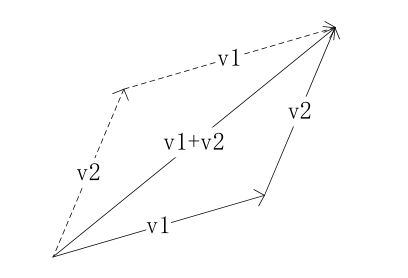向量的减法代表另一条对角线（）时，叫做被减向量，叫做减向量，记方向的一个口决是：指向被减向量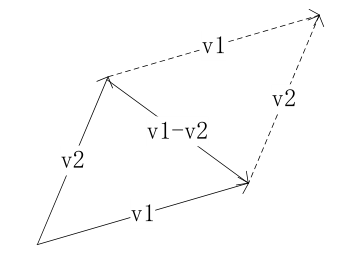【向量的点积】
向量点积的定义，拿三维向量来说：

这里要注意向量的点积结果是一个数值，而非一个向量。
下面来推导点积的最重要的几何意义公式其中是两个向量之间的夹角。当和都是单位向量时， 这是图形学界使用频率最高的公式之一。仅对二三维有效。
下面来进行推导：
设，它们的终点分别为,
那么，在三角形OAB中，使用余弦定理：
其中是与的夹角。
将A,B,的值代入后，整理即得
【向量的叉积】
先看叉积的性质。两个向量的叉积的结果是垂直于这两个向量和的第三个向量，它的方向使用右手螺旋定则，长度则为和所围的平行四边形的面积。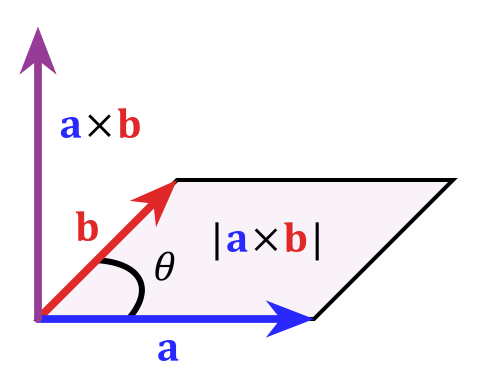，其中为和的夹角。
叉乘满足的基本性质如下：
，自己与自己的夹角=0，则=0，所夹的平行四边形的面积也是0。
再看叉乘在数值计算上的定义：

(展开式一）
先讨论正交基的运算法则：

再代入到（展开式一）：

【计算正交基】
向量的经常的一个操作是构造正交基，根据一个向量，构建正交基，往往需要用到叉乘。在给定的单位向量，构建一个与之垂直的向量，则保证其相互垂直，则，随手可构造，再构造
在pbrt-v2的geometry.h的针对向量的定义中有个内联函数用来根据一个输入的向量，计算正交基，输入一个v1，输出v2, 和v3,都是单位向量：
inline void CoordinateSystem(const Vector &v1, Vector *v2, Vector *v3) {
if (fabsf(v1.x) > fabsf(v1.y)) {
float invLen = 1.f / sqrtf(v1.x*v1.x + v1.z*v1.z);
*v2 = Vector(-v1.z * invLen, 0.f, v1.x * invLen);
}
else {
float invLen = 1.f / sqrtf(v1.y*v1.y + v1.z*v1.z);
*v2 = Vector(0.f, v1.z * invLen, -v1.y * invLen);
}
*v3 = Cross(v1, *v2);
}


展开全文向量叉乘 向量点乘 正交基
• 数量积即点积。 定义 在数学中，数量积（dot product; scalar product，也称为标量积、点积、点乘）是接受在实数R上的两个矢量并返回一个实数值标量的二元运算。它是欧几里得空间的标准内积。 两个矢量a = [a1, ...


点积
数量积即点积。
定义
在数学中，数量积（dot product; scalar product，也称为标量积、点积、点乘）是接受在实数R上的两个矢量并返回一个实数值标量的二元运算。它是欧几里得空间的标准内积。
两个矢量a = [a1, a2,…, an]和b = [b1, b2,…, bn]的点积定义为：
a·b=a1b1+a2b2+……+anbn
使用矩阵乘法并把（纵列）矢量当作n×1 矩阵，点积还可以写为：
a·b=a^T*b，这里的a^T指示矩阵a的转置。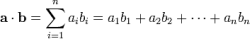点积的值由以下三个值确定：u的大小、v的大小、u,v夹角的余弦。在u,v非零的前提下，点积如果为负，则u,v形成的角大于90度；如果为零，那么u,v垂直；如果为正，那么u,v形成的角为锐角。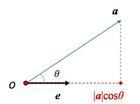点积
点积得到两个向量的夹角的cos值，通过它可以知道两个向量的相似性，利用点积可判断一个多边形是否面向摄像机还是背向摄像机
向量的点积与它们夹角的余弦成正比，因此在聚光灯的效果计算中，可以根据点积来得到光照效果，如果点积越大，说明夹角越小，则物理离光照的轴线越近，光照越强。运算律
1.交换律：α·β=β·α　2.分配律：(α+β)·γ=α·γ+β·γ　3.若λ为数：(λα)·β=λ(α·β)=α·(λβ)　若λ、μ为数：：(λα)·(μβ)=λμ(α·β)　4.α·α=|α|^2 ，此外：α·α=0〈=〉α=0。　向量的数量积不满足消去律，即一般情况下：α·β=α·γ，α≠0 ≠〉β=γ。　向量的数量积不满足结合律，即一般（α·β)·γ ≠〉α·（β·γ）　相互垂直的两向量数量积为0
坐标表示
已知两个非零向量a=（x1，y1），b=（x2，y2），则有a·b=x1x2+y1y2，即两个向量的数量积等于它们对应坐标的乘积的和。
应用
平面向量的数量积a·b是一个非常重要的概念，利用它可以很容易地证明平面几何的许多命题，例如勾股定理、菱形的对角线相互垂直、矩形的对角线相等等　如证明勾股定理：　Rt△ABC中，∠C=90°，则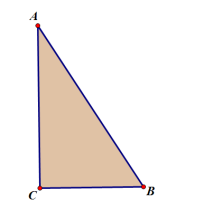|CA|^2+|CB|^2=|AB|^2：　因AB = CB-CA，
所以AB·AB =（CB-CA）·（CB-CA）= CB·CB-2CA·CB+CA·CA;　由∠C=90°，有CA⊥BD，于是CA·CB=0　所以AB·AB=AC·AC+CB·CB　菱形对角线相互垂直：　菱形ABCD中,点O为对角线AC、BD的交点，求证AC⊥BD　设|AB|=|BC|=|CD|=|DA|=a　因AC=AB+BC;BD=BC+CD
所以AC·BD=(AB+BC)(BC+CD)=a^2(2cosα+2cosπ-α ）　又因为cosα=-cosπ-α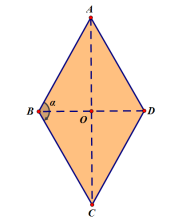所以AC·BD=(AB+BC)(BC+CD)=a^2(2cosα+2cosπ-α ）=0　AC⊥B
D
在生产生活中，点积同样应用广泛。利用点积可判断一个多边形是否面向摄像机还是背向摄像机。向量的点积与它们夹角的余弦成正比，因此在聚光灯的效果计算中，可以根据点积来得到光照效果，如果点积越大，说明夹角越小，则物理离光照的轴线越近，光照越强。物理中，点积可以用来计算合力和功。若b为单位矢量，则点积即为a在方向b的投影，即给出了力在这个方向上的分解。功即是力和位移的点积。计算机图形学常用来进行方向性判断，如两矢量点积大于0，则它们的方向朝向相近；如果小于0，则方向相反。矢量内积是人工智能领域中的神经网络技术的数学基础之一，此方法还被用于动画渲染（Animation-Rendering）。
向量积
向量积 也被称为矢量积、叉积（即交叉乘积）、外积，是一种在向量空间中向量的二元运算。与点积不同，它的运算结果是一个伪向量而不是一个标量。并且两个向量的叉积与这两个向量垂直。
定义
两个向量a和b的叉积写作a×b（有时也被写成a∧b，避免和字母x混淆）。向量积可以被定义为：
|向量a×向量b|=|a||b|sinθ在这里θ表示两向量之间的角夹角（0° ≤ θ ≤ 180°），它位于这两个矢量所定义的平面上。
这个定义有一个问题，就是同时有两个单位向量都垂直于和：若满足垂直的条件，那么也满足。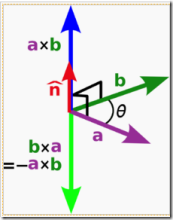一个简单的确定满足“右手定则”的结果向量的方向的方法是这样的：若坐标系是满足右手定则的，当右手的四指从a以不超过180度的转角转向b时，竖起的大拇指指向是c的方向。
向量积|c|=|a×b|=|a| |b|sin<a,b>
即c的长度在数值上等于以a，b，夹角为θ组成的平行四边形的面积。
c的方向垂直于a与b所决定的平面，c的指向按右手规则从a转向b来确定。
a×b=(aybz-azby)i+(azbx-axbz)j+(axby-aybx)k,为了帮助记忆，利用三阶行列式，写成
| i j k|
|ax ay az|
|bx by bz|
b×a= -a×b右手规则
三角形ABC的面积=1/2*abs(AB×AC)
性质
几何意义
叉积的长度 |a×b| 可以解释成以a和b为邻边的平行四边形的面积。
混合积 [a b c] = (a×b)·c可以得到以a，b，c为棱的平行六面体的体积。
代数规则
反交换律：
a×b= -b×a
加法的分配律：
a× (b+c) =a×b+a×c
与标量乘法兼容：
(ra) ×b=a× (rb) = r(a×b)
不满足结合律，但满足雅可比恒等式：
a× (b×c) +b× (c×a) +c× (a×b) =0
分配律，线性性和雅可比恒等式别表明：具有向量加法和叉积的 R3 构成了一个李代数。
两个非零向量 a 和 b 平行，当且仅当a×b=0
拉格朗日公式
这是一个著名的公式，而且非常有用：
a× (b×c) =b(a·c) -c(a·b),
证明过程如下：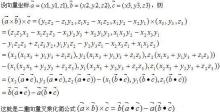二重向量叉乘化简公式及证明
可以简单地记成“BAC - CAB”。这个公式在物理上简化向量运算非常有效。需要注意的是，这个公式对微分算子不成立。
这里给出一个和梯度相关的一个情形：
这是一个霍奇拉普拉斯算子的霍奇分解 的特殊情形。
另一个有用的拉格朗日恒等式是：
这是一个在四元数代数中范数乘法 | vw | = | v | | w | 的特殊情形。
矩阵形式
给定直角坐标系的单位向量i，j，k满足下列等式：
i×j=k；
j×k=i ；
k×i=j ；
通过这些规则，两个向量的叉积的坐标可以方便地计算出来，不需要考虑任何角度：设
a= [a1, a2, a3] =a1i+ a2j+ a3k
b= [b1,b2,b3]=b1i+ b2j+ b3k ;
则
a × b= [a2b3-a3b2,a3b1-a1b3, a1b2-a2b1]
上述等式可以写成矩阵的行列式的形式：
叉积也可以用四元数来表示。注意到上述 i，j，k 之间的叉积满足四元数的乘法。一般而言，若将向量 [a1, a2, a3] 表示成四元数 a1i + a2j + a3k，两个向量的叉积可以这样计算：计算两个四元数的乘积得到一个四元数，并将这个四元数的实部去掉，即为结果。更多关于四元数乘法，向量运算及其几何意义请参见四元数与空间旋转。
高维情形
七维向量的叉积可以通过八元数得到，与上述的四元数方法相同。
七维叉积具有与三维叉积相似的性质：
双线性性：
x × (ay + bz) = ax × y + bx × z
(ay + bz) × x = ay × x + bz × x.
反交换律：
x × y + y × x = 0
同时与 x 和 y 垂直：
x · (x × y) = y · (x × y) = 0
拉格朗日恒等式
|x × y|2 = |x|2 |y|2 - (x · y)2.
不同于三维情形，它并不满足雅可比恒等式：
x × (y × z) + y × (z × x) + z × (x × y) ≠ 0
应用
在物理学光学和计算机图形学中，叉积被用于求物体光照相关问题。
求解光照的核心在于求出物体表面法线，而叉积运算保证了只要已知物体表面的两个非平行矢量(或者不在同一直线的三个点),就可依靠叉积求得法线。

展开全文叉积
• 向量积，数学中又称外、叉积，物理中称矢、叉乘，是一种在向量空间中向量的二元运算。与点不同，它的运算结果是一个向量而不是一个标量。并且两个向量的叉积与这两个向量和垂直。其应用也十分广泛，通常应用于...
向量积，数学中又称外积、叉积，物理中称矢积、叉乘，是一种在向量空间中向量的二元运算。与点积不同，它的运算结果是一个向量而不是一个标量。并且两个向量的叉积与这两个向量和垂直。其应用也十分广泛，通常应用于物理学光学和计算机图形学中。 表示方法 两个向量a和b的叉积写作a×b（有时也被写成a∧b，避免和字母x混淆）。 定义 向量积可以被定义为：|a×b|=|a||b|sin<a，b>。 模长：（在这里θ表示两向量之间的夹角（共起点的前提下）（0°≤θ≤180°），它位于这两个矢量所定义的平面上。）
方向：a向量与b向量的向量积的方向与这两个向量所在平面垂直，且遵守右手定则。（一个简单的确定满足“右手定则”的结果向量的方向的方法是这样的：若坐标系是满足右手定则的，当右手的四指从a以不超过180度的转角转向b时，竖起的大拇指指向是c的方向。） 也可以这样定义（等效）： 向量积|c|=|a×b|=|a||b|sin<a，b> 即c的长度在数值上等于以a，b，夹角为θ组成的平行四边形的面积。 而c的方向垂直于a与b所决定的平面，c的指向按右手定则从a转向b来确定。
几何意义及其运用 叉积的长度|a×b|可以解释成这两个叉乘向量a，b共起点时，所构成平行四边形的面积。据此有：混合积[abc]=（a×b）·c可以得到以a，b，c为棱的平行六面体的体积。
与数量积的区别 注：向量积≠向量的积（向量的积一般指点乘） 一定要清晰地区分开向量积（矢积）与数量积（标积）。 　　 名称： 标积/内积/数量积/点积  运算式：（a，b和c粗体字，表示向量） a·b=|a||b|·cosθ  几何意义 ：向量a在向量b方向上的投影与向量b的模的乘积 运算结果： 标量（常用于物理）/数量（常用于数学）
来源（https://baike.baidu.com/item/%E5%90%91%E9%87%8F%E7%A7%AF/4601007?fr=aladdin）
展开全文叉积...